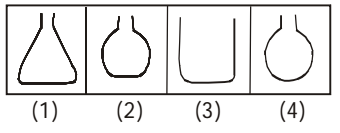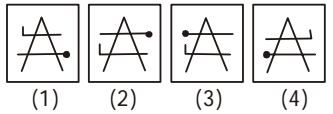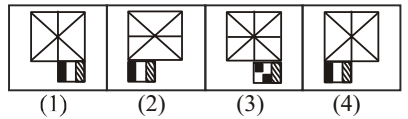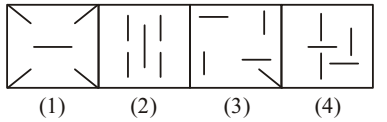## Classification

#### Classification

Direction: Select the one which is different from the other three responses.

1. NA1. Except figure (3), in all other figures have small opening.

##### Correct Option: C

All other figures have small opening. But figure (3) is different to others .

1. NA1. Except one figure , in all other figures the arrow is pointing clockwise.

##### Correct Option: A

Except in figure (1), in all other figures the arrow is pointing clockwise. So , figure ( 1 ) is correct .

1. NA1. Except one figure , all other figures the line segments point differently.

##### Correct Option: C

Except in figure (3), all other figures the line segments point differently.

1. NA1. In figure (3) the orientation of lower design is different.

##### Correct Option: C

In figure (3) the orientation of lower design is different.So , figure (3) is odd .

Direction: Select the odd figure from the given responses.

1. NA1. Except one figure, in all other figures all the five segments are of equal length.

##### Correct Option: B

Except in figure (2), in all other figures all the five segments are of equal length.